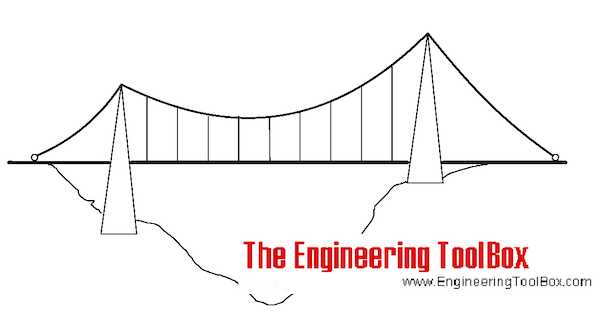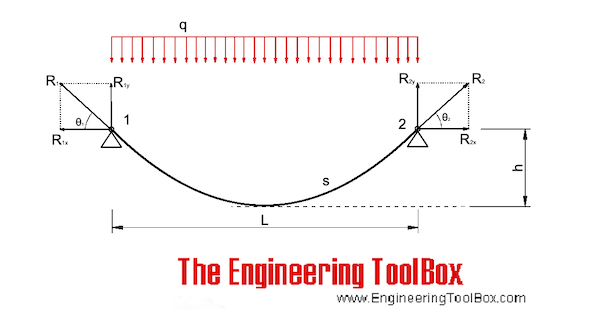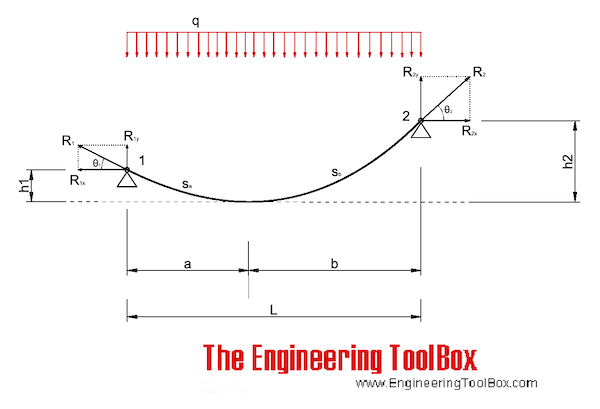Engineering ToolBox - Resources, Tools and Basic Information for Engineering and Design of Technical Applications!

## Force and tension in cables with uniform loads.The equations below can also be used for cables only loaded with their own weight as long as the sagging height (h) vs. length (L) ratio is below 0.1.The cable follows the shape of a parable and the horizontal support forces can be calculated as

R1x = R2x

= q L2 / (8 h)                               (1)

where

R1x = R2x = horizontal support forces (lb, N) (equal to midspan lowest point tension in cable)

q = unit load (weight) on the cable (lb/ft, N/m)

L = cable span (ft, m)

h = cable sag (ft, m)

The vertical support forces at the end of the cable can be calculated as

R1y = R2y

= q L / 2                               (1a)

where

R1y = R2y = vertical support forces  (lb, N)

The resultant forces acting in the end supports - and in the direction of the cable close to the supports - can be calculated as

R1 = R2

= (R1x2 + R1y2)0.5

= (R2x2 + R2y2)0.5                               (1b)

where

R1,2 = resultant force at the support (lb, N)

The angle θ can be calculated as

θ = tan-1(R1y / R1x)

= tan-1(R2y / R2x)           (1c)

The length of the sagged cable can be approximated to

s = L + 8 h2 / (3 L)                                 (1d)

where

s = cable length (ft, m)

Note that the equation is not valid when h > L / 4.

• kip = 1000 lb
• klf = kip per linear foot

q - uniform load (N/m, lb/ft)

L - length (m, ft)

h - sag (m, ft)

R12x (N, lb): 45
R12y (N, lb): 60
R12 (N, lb): 75
θ (degrees): 53.1
s (m, ft):

#### Example - Uniform Cable Load, Imperial units

A cable with length 100 ft and a sag 30 ft has a uniform load 850 lb/ft. The horizontal supports and mid-span cable forces can be calculated as

R1x = R2x

= (850 lb/ft) (100 ft)2 / (8 (30 ft))

= 35417 lb

The vertical forces at the supports can be calculated as

R1y = R2y

= (850 lb/ft) (100 ft) / 2

=  42500 lb

The resultant forces acting in the supports can be calculated as

R1,2 = ((35417 lb)2 + (42500 lb)2)0.5

= 55323 lb

The angle θ can be calculated as

θ = tan-1((42500 lb) / (35417 lb))

= 50.2 o

The length of the sagged cable can be approximated to

s = (100 ft) + 8 (30 ft)2 / (3 (100 ft))

= 124 ft

#### Example - Uniform Cable Load, SI units

A cable with length 30 m and a sag 10 m has a uniform load of 4 kN/m. The horizontal supports and mid-span cable forces can be calculated as

R1x = R2x

= (4000 N/m) (30 m)2 / (8 (10 m))

= 45000 N

= 45 kN

The vertical support forces can be calculated as

R1y = R2y

= (4000 N/m) (30 m) / 2

=  60000 N

= 60 kN

The angle θ can be calculated as

θ = tan-1((60 kN) / (45 kN))

= 53.1 o

The resultant force acting in the supports can be calculated as

R1,2 = ((45000 N)2 + (60000 N)2)0.5

= 75000 N

= 75 kN

The length of the sagged cable can be approximated to

s = (30 m) + 8 (10 m)2 / (3 (30 m))

= 38.9 m

#### Example - Known Tension at the Supports - Calculate Sagging and Length of Cable

For a 30 m long cable with uniform load 4 kN/m the resulting tension in the cable at the end supports are 100 kN.

The vertical forces in the supports can be calculated as

R1y = R2y

= (4 kN/m) (30 m) / 2

= 60 kN

The horizontal forces in the supports can be calculated as

R1x = R2x

= ((100 kN)2 - (60 kN)2)0.5

= 80 kN

The angle θ can be calculated as

θ = tan-1((60 kN) / (80 kN))

= 36.9 o

The sagging can be calculated by modifying eq.1 to

h = q L2 / (8 R1x)

= (4 kN/m) (30 m)2 / (8 (80 kN))

= 5.6 m

The length of the sagged cable can be estimated to

s = (30 m) + 8 (5.6 m)2 / (3 (30 m))

= 32.8 m

### Uniformly Loaded Cables with Inclined Chords#### Inclined Cable Calculator - with Uniform Horizontal Loads

The calculator below can be used for cables with inclined chords and uniformly loads. The calculator is based on an iterative algorithm where the parable shaped cable is adapted to span L, height h1 and h2 according the figure above. The parable equation estimated below can be used to replicate the shape in spreadsheets or CAD systems.

inputs
canvas
results

The horizontal support forces in x-direction can be calculated as

R1x = R2x

= q a2 / (2 h1)

= q b2 / (2 h2)                            (2a)

If b > a the maximum forces in the cable and at support 1 and 2 can be calculated as

R2 = (R2x2 + (q b)2)0.5                   (2c)

R1 = (R1x2 + (q a)2)0.5                   (2d)

- and the vertical forces at support 1 and 2 can be calculated as

R2y = (R22 - R2x2)0.5                    (2e)

R1y = (R12 - R1x2)0.5                    (2f)

The angles between the horizontal and resulting forces can be calculated as

θ2 = cos-1(R2x / R2)                    (2g)

θ1 = cos-1(R1x / R1)                   (2g)

The length of the sagged cable can be estimated as

sb = b (1 + 2/3 (h2 / b)2)             (2h)

sa = a (1 + 2/3 (h1 / a)2)             (2i)

s = sa + sb                                (2j)

#### Example - Inclined Cable with Uniform Load, SI units

A cable with span 30 m, length a = 7.2 m, length b = 22.8 m, sag h1= 1 m and sag h2 = 10 m has a uniform load of 4 kN/m.

The horizontal support forces can be calculated as

R1x = R2x

= (4 kN/m) (30 m)2 / (2 (((1 m))0.5 + ((10 m))0.5))

= 104 kN

The resulting support forces can be calculated as

R2 = ((103.9 kN)2 + ((4 kN/m) (22.8 m))2)0.5

= 138 kN

R1 = ((103.9 kN)2 + ((4 kN/m) (7.2 m))2)0.5

= 108 kN

The vertical forces in the supports can be calculated as

R2y = ((138.2 kN)2 - (103.9 kN)2)0.5

=  91.2 kN

R1y((107.8 kN)2 - (103.9 kN)2)0.5

= 28.8 kN

The angles between resulting and horizontal forces in support 1 and 2 can be calculated as

θ2 = cos-1((103.9 kN)/ (138.2 kN))

= 41.3 o

θ1 = cos-1((103.9 kN)/ (107.8 kN))

= 15.5 o

The length of the sagged cable can be calculated as

sb = (22.8 m) (1 + 2/3 ((10 m) / (22.8 m))2

= 25.7 m

sa (7.2 m) (1 + 2/3 ((1 m) / (7.2 m))2)

= 7.3 m

s = (7.3 m) + (25.7 m )

= 33 m

## Related Topics

• ### Mechanics

Forces, acceleration, displacement, vectors, motion, momentum, energy of objects and more.
• ### Statics

Loads - forces and torque, beams and columns.

## Related Documents

• ### Forces and Tensions in Ropes due to Angle

Reduced load capacities in ropes, cables or lines - due to acting angle.
• ### Manila Ropes - Strength

Minimum breaking strength and safe load of manila 3-strand rope.
• ### Polyester Polyolefin Ropes - Strengths

Polyester Polyolefin dual fiber 3-strand rope - minimum breaking strength and safe load.
• ### Polyester Ropes - Strengths

Polyester 3-strand and 8-strand rope - minimum breaking strength and safe load.
• ### Polypropylene Fiber Ropes - Strengths

Polypropylene fiber 3-strand and 8 strand rope - minimum breaking strength and safe load.

• ### Sisal Ropes - Strengths

Sisal rope 3-strand, minimum breaking strength and safe load.
• ### Wire Rope Slings

Sling angles and influence on capacity.

## Engineering ToolBox - SketchUp Extension - Online 3D modeling!

Add standard and customized parametric components - like flange beams, lumbers, piping, stairs and more - to your Sketchup model with the Engineering ToolBox - SketchUp Extension - enabled for use with older versions of the amazing SketchUp Make and the newer "up to date" SketchUp Pro . Add the Engineering ToolBox extension to your SketchUp Make/Pro from the Extension Warehouse !

We don't collect information from our users. More about

## Citation

• The Engineering ToolBox (2012). Cable Loads. [online] Available at: https://www.engineeringtoolbox.com/cable-loads-d_1816.html [Accessed Day Month Year].

Modify the access date according your visit.

9.19.12